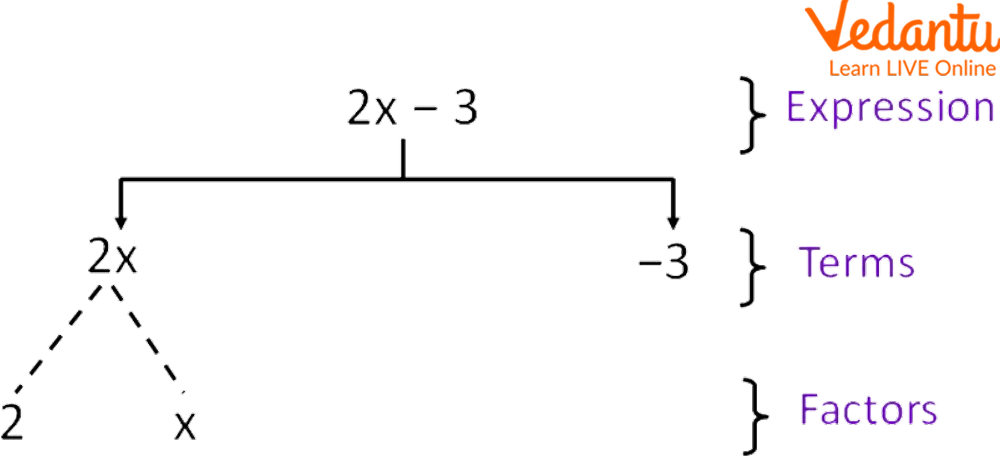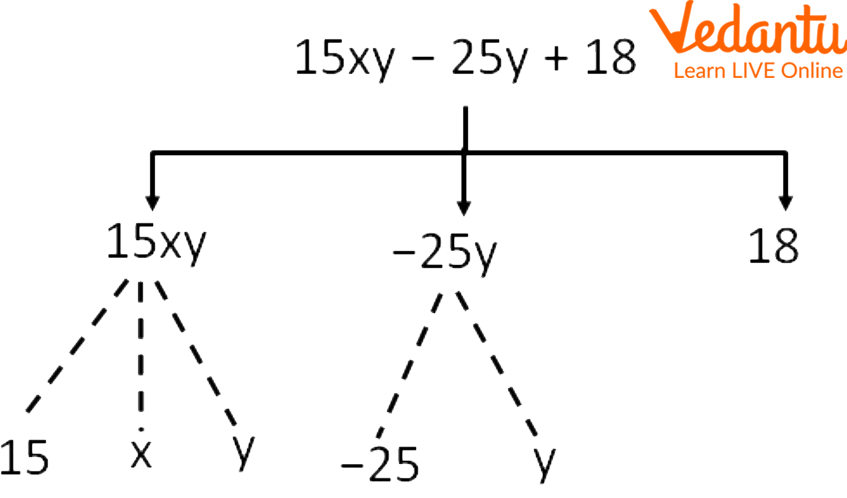Courses
Courses for Kids
Free study material
Free LIVE classes
More

# Terms and Factors of an ExpressionLIVE
Join Vedantu’s FREE Mastercalss

## Overview of the Factors of an Expression

Have you ever thought, about what the terms with the same algebraic factors are called? The term 'factor' is used to express a number as the produt of any two numbers. Factorization is a method for determining factors for any mathematical object, such as a number, a polynomial, or an algebraic expression. Thus, factorization of an algebraic expression refers to the process of identifying the factors of a given algebraic expression. In the given article, children would gain knowledge about the factors of the terms of an expression and the way how to write the factors. Reading this article, students will be able to identify the factors of the terms of the expression. This is the most common topic of algebra, thus should be on the tips of every child to excel in advanced mathematics.

## What are the Factors of a Term of an Expression?

The numbers and variables that are multiplied together to form a term are called the factors of the term. For example, 5xyz is a term, whose factors are 5, x, y, and z. Factors can either be positive or negative, but not zero. One cannot further factorize the factors. Generally, factors have a wide application in daily life.Terms and Factors of an Expression

Factors can be used to divide the entire term by leaving the remainder zero. The factor of the expression is also called the coefficient. The terms with the same algebraic factors are called like terms. For eg: 8y + 3y. While the terms with varied algebraic factors are called, unlike terms. For eg: 2x + 6y.

## How to Find Factors?

Steps to find the factors of the term of the expression using the tree diagram are discussed below:

1. Write the given expression

2. Separate each term by removing the arithmetical operations and write just below the given expression

3. Then, factorize the term i.e. writing all the numbers and variables separately

## How to Write Factors?

Before we proceed with the topic; of how to write factors, one should be aware of the way to identify the factors of the terms of the expression. The way of writing factors is very simple. Students just need to write all the numbers or variables being written in terms separately by putting commas in between.

## Solved Examples

Q 1. Find the factors of the algebraic expression \$2+x+x^2\$.

Ans: Using a tree diagram,

1. Write the given expression

\$2+x+x^2\$

1. Separate the terms of the given expression, as

\$2, x, x^2\$

1. Write corresponding factors to each term of the expression

\$2, x, x, x\$Factors of the Given Expression

Q 2. What are the terms and factors in the expression?

15xy – 25y + 18

Ans: Using a tree diagram,

1. Write the given expression

15xy – 25y + 18

1. Separate the terms of the given expression, as

15xy, -25y, 18

1. Write corresponding factors to each term of the expression

15, x, y, -25, y, 18Showing Factors of the Expression, 15xy – 25y + 18

## Practice Problems

Q 1. Discover the factors of the number 108.

Ans: 1, 2, 3, 4, 6, 9, 12, 18, 27, 36, 54, and 108

Q 2. Identify the factors of the given expression

3x2 - 2

Ans: 3, x, x, -2

Q 3. Identify the terms of the algebraic expression 7x – 9.

Ans: 7x and 9

Q 4. Write the terms in the expression: 9x – 7y + 5 and write the corresponding factors to each term.

Ans: Terms are 9x, -7y, and 5.

Factors are 9, x, -7, y, 5.

## Summary

Summing up here with the concept of factors of an expression and the way to find these factors. Every topic in this article has been discussed in a simple language and attractive format for a better understanding of the concepts. Some solved examples are also discussed to make students master the given topics. Practice problems are given above that need to be solved by the students themselves. Hoping you enjoyed reading it. Feel free to ask your doubts by writing in the comments section given below.

Last updated date: 20th Sep 2023
Total views: 94.5k
Views today: 2.94k

## FAQs on Terms and Factors of an Expression

1. What is an algebraic expression?

An algebraic expression is the combination of variables and constants connected using the arithmetic operations such as +, -, ×, ÷. For example x2 - 2y + 1.

2. Define a term.

A term can be defined as a single number, variable, the product of two variables, or the product of a number and a variable. For example 2y, 5t, 9, etc. An algebraic expression is formed using different terms together.

3. What are the different types of polynomials?

There are three different types of polynomials:

• Monomial: A Polynomial that has only one term. For eg: 5x.
• Binomial: Polynomials that have two terms. For eg: 4x+5y.
• Trinomial: Polynomials that have three terms. For eg: 3x+4y+5z.